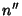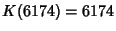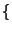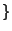## Kaprekar Routine

A routine discovered in 1949 by D. R. Kaprekar for 4-digit numbers, but which can be generalized to-digit numbers. To apply the Kaprekar routine to a number, arrange the digits in descending () and ascending () order. Now computeand iterate. The algorithm reaches 0 (a degenerate case), a constant, or a cycle, depending on the number of digits inand the value of.

For a 3-digit numberin base 10, the Kaprekar routine reaches the number 495 in at most six iterations. In base, there is a unique numberto whichconverges in at mostiterations Iffis Even. For any 4-digit numberin base-10, the routine terminates on the number 6174 after seven or fewer steps (where it enters the 1-cycle).

2. 0, 0, 9, 21,(45), (49), ...,

3. 0, 0, (32, 52), 184, (320, 580, 484), ...,

4. 0, 30,201, (126, 138), (570, 765),(2550), (3369), (3873), ...,

5. 8, (48, 72), 392, (1992, 2616, 2856, 2232), (7488, 10712, 9992, 13736, 11432), ...,

6. 0, 105, (430, 890, 920, 675, 860, 705),5600, (4305, 5180),(27195), (33860), (42925), (16840, 42745, 35510), ...,

7. 0, (144, 192), (1068, 1752, 1836), (9936, 15072, 13680, 13008, 10608), (55500, 89112, 91800, 72012, 91212, 77388), ...,

8. 21, 252,(1589, 3178, 2723), (1022, 3122, 3290, 2044, 2212),(17892, 20475), (21483, 25578, 26586, 21987), ...,

9. (16, 48), (320, 400),(2256, 5312, 3856), (3712, 5168, 5456),41520, (34960, 40080, 55360, 49520, 42240), ...,

10. 0, 495, 6174,(53955, 59994), (61974, 82962, 75933, 63954), (62964, 71973, 83952, 74943), ...,

References

Eldridge, K. E. and Sagong, S. The Determination of Kaprekar Convergence and Loop Convergence of All 3-Digit Numbers.'' Amer. Math. Monthly 95, 105-112, 1988.

Kaprekar, D. R. An Interesting Property of the Number 6174.'' Scripta Math. 15, 244-245, 1955.

Trigg, C. W. All Three-Digit Integers Lead to...'' The Math. Teacher, 67, 41-45, 1974.

Young, A. L. A Variation on the 2-digit Kaprekar Routine.'' Fibonacci Quart. 31, 138-145, 1993.